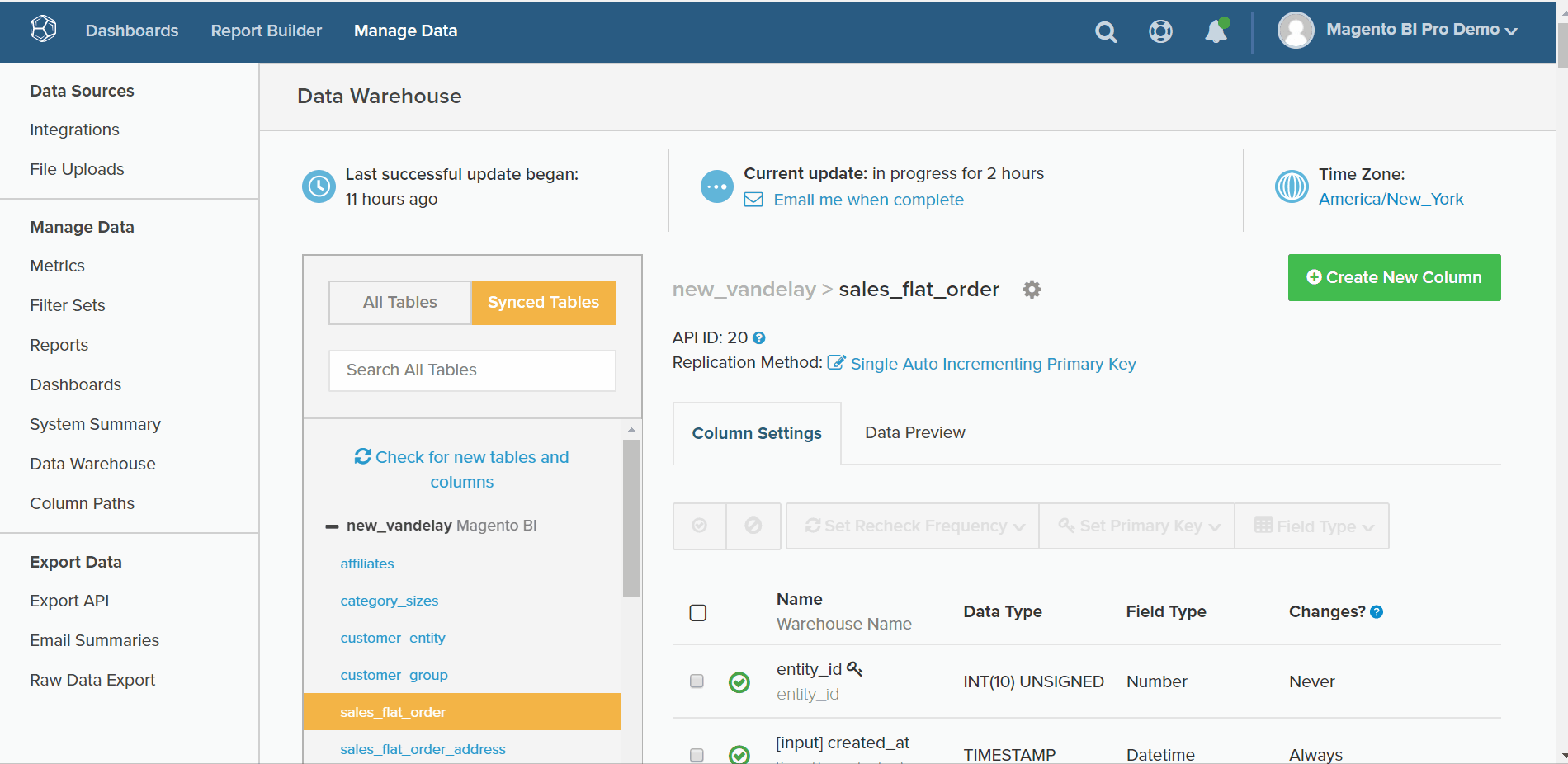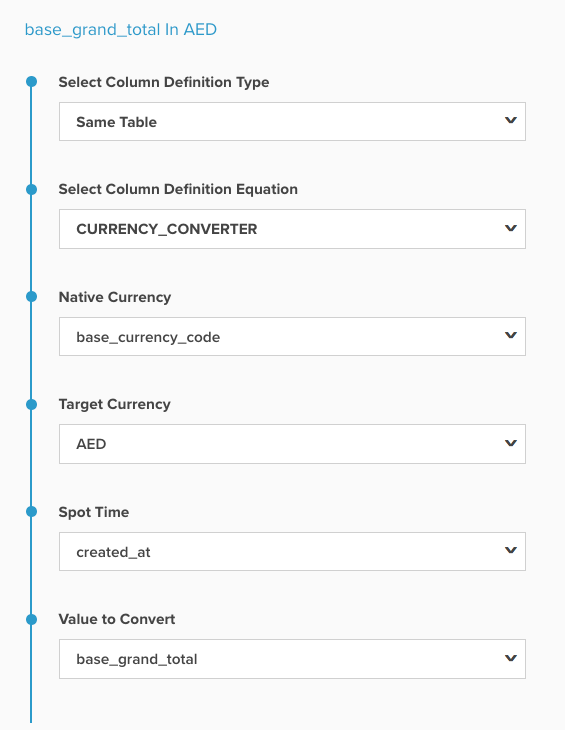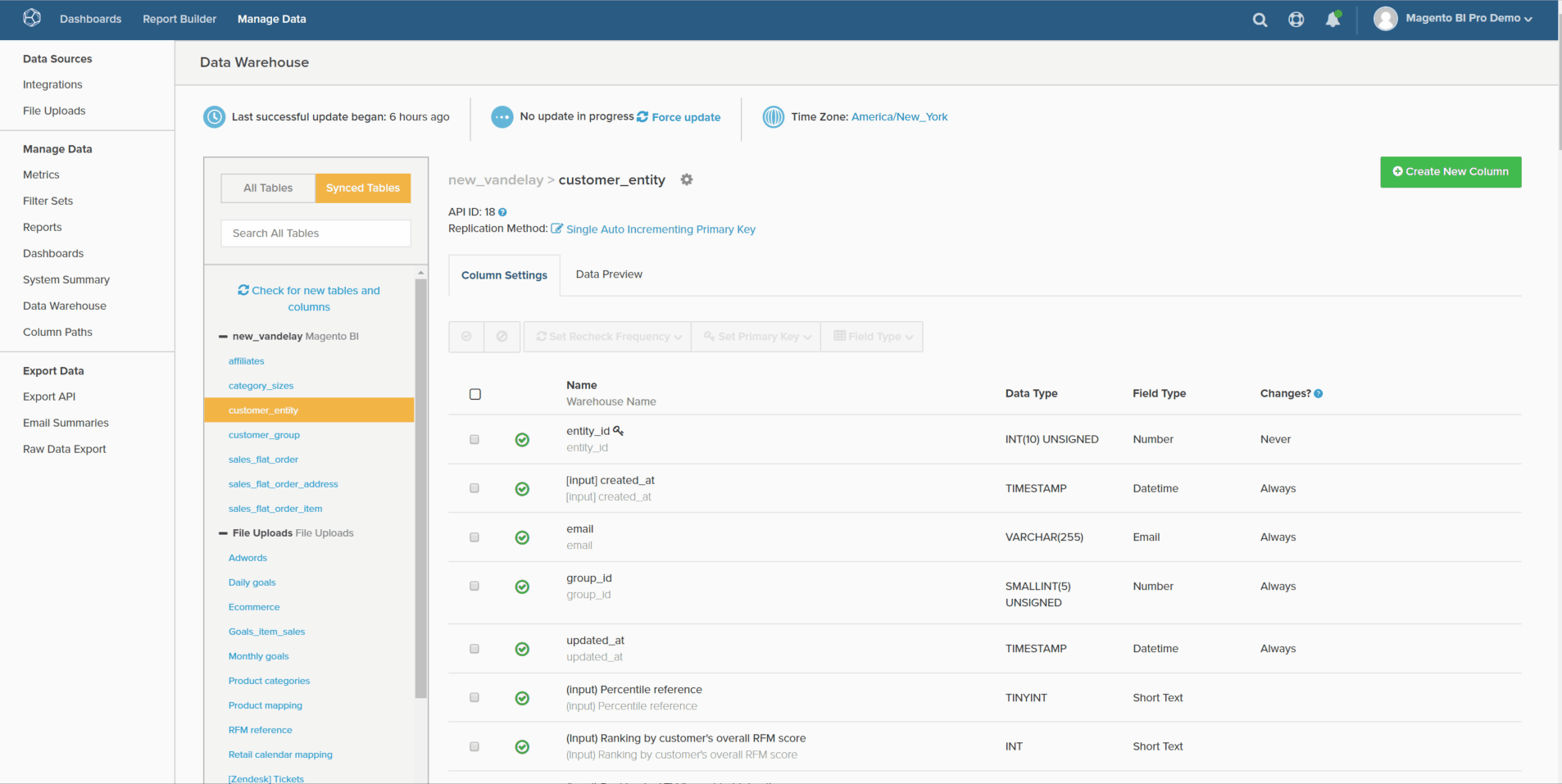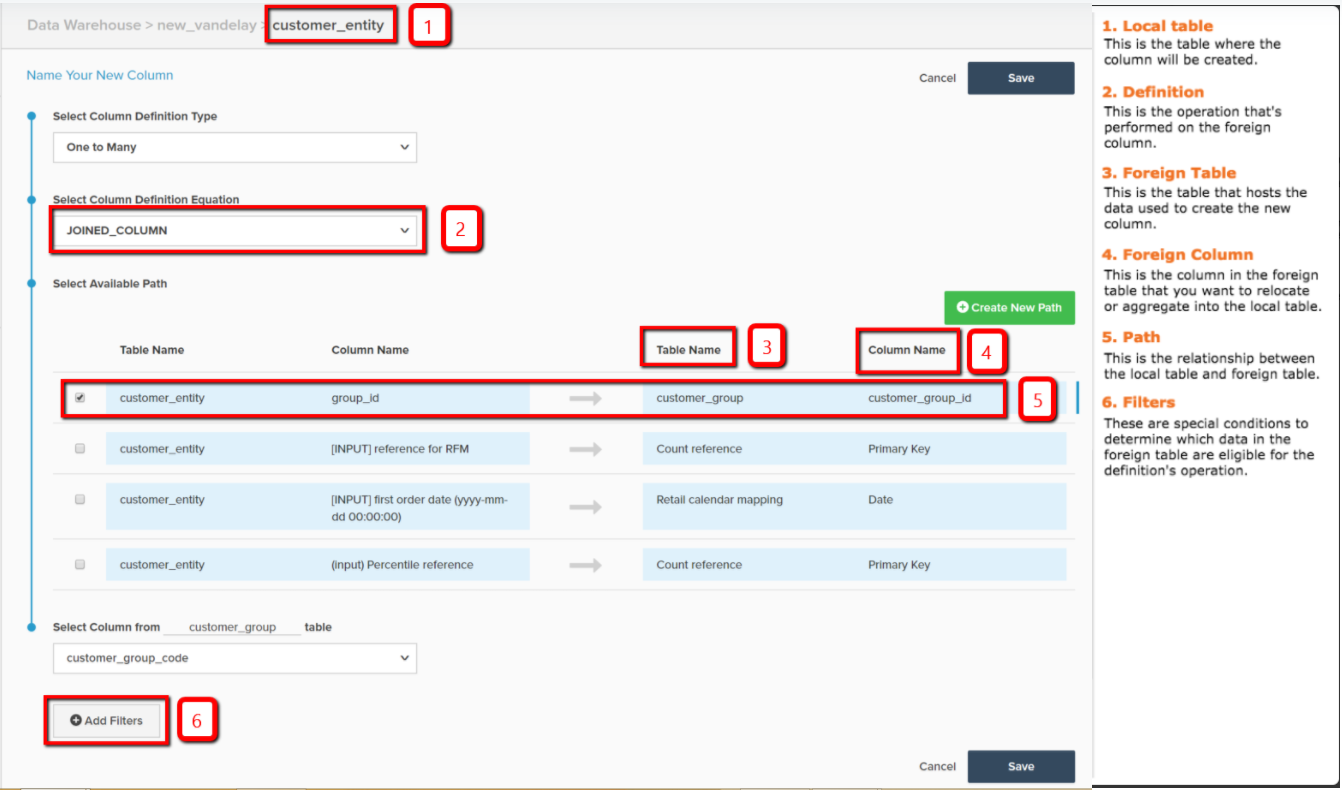# Calculated Column Types

Within the Data Warehouse Manager, you can create columns to augment and optimize your data for analysis. This functionality can be accessed by selecting any table in the Data Warehouse Manager and clicking Create New Column.

This topic describes the types of columns that you can create with the Data Warehouse Manager. It also covers the description, a visual walk-through of that column, and a reference map of all the inputs required to create a column. There are three ways to create calculated columns:

## Same table calculated columns

These columns are built using input columns from the same table.

### Age

An age calculated column returns the number of seconds between the current time and some input time.

The example below creates `Seconds since customer's most recent order` in the `customers` table. This can be used to construct user lists of customers who have not made purchases (sometimes referred to as churning) within `X days`.### Currency Converter

A currency converter calculated column converts the native currency of a column to a desired new currency.

The example below creates `base\_grand\_total In AED`, converting the `base\_grand\_total` from it is native currency to AED in the `sales\_flat\_order` table. This column works well for stores with multiple currencies that want to report in their local currency.

For Commerce clients, the `base\_currency\_code` field typically stores native currencies. The `Spot Time` field should match the date used in your metrics.## One-to-many calculated columns

`One-to-Many` columns use a path between two tables. This path always implies a one table, where an attribute lives, and a many table, where that attribute gets “relocated” down to. The path can be described as a `foreign key--primary key` relationship.

### Joined column

A joined column relocates an attribute on the one table to the many table. The classic example of one/many is customers (one) and orders (many).

In the example below, the `Customer's group\_id` dimension gets joined down into the `orders` table.## Many-to-one calculated columns

These columns use the same paths that one-to-many columns do, but they point data in the opposite direction. The column gets created on the one side of the path, as opposed to the many side. Because of this relationship, the value in the column needs to be an aggregation, that is, a mathematical operation performed on the data points on the many side. There are many use cases for this, and a few are listed below.

### Count

This type of calculated column returns the count of values on the many table onto the one table.

In the example below, the dimension `Customer's lifetime number of canceled orders` is created on the `customers` table (with a filter for `orders.status`).### Sum

A sum calculated column is the sum of values on the `many` table onto the one table.

This can be used to create customer-level dimensions like `Customer's lifetime revenue`.

### Min or Max

A min or max calculated column returns the smallest or largest record that exists on the many side.

This can be used to create customer-level dimensions like `Customer's first order date`.

### Exists

An calculated column is a binary test determining the presence of a record on the many side. In other words, the new column returns a `1` if the path connects at least one row in each table, and `0` if no connection can be made.

This type of dimension might determine, for example, if a customer ever purchased a particular product. Using a join between a `customers` table and `orders` table, a filter for a specific product, a dimension `Customer has purchased Product X?` can be built.

## Handy reference map

If you are having trouble remembering what all the inputs are when creating a calculated column, keep this reference map handy when you are building: